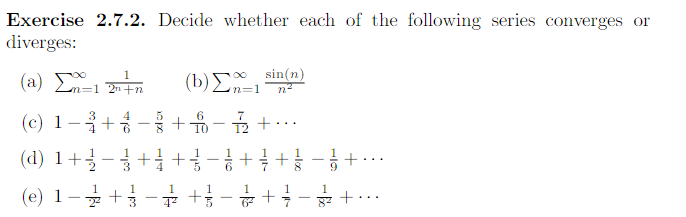Home / Answered Questions / Other / exercise-2-7-2-decide-whether-each-of-the-following-series-converges-or-diverges-a-l-1-b-an-1-sinkn--aw580

# (Solved): Exercise 2.7.2. Decide Whether Each Of The Following Series Converges Or Diverges: (a) L=1 *** (b) A...Exercise 2.7.2. Decide whether each of the following series converges or diverges: (a) L=1 *** (b) An=1 sinkn) (c) 1-Ã¥+\$ - \$ + - +... (d) 1+* - *+*+*-* +++ } - + ... (e) 1-3 +}- +- + - +...

We have an Answer from Expert